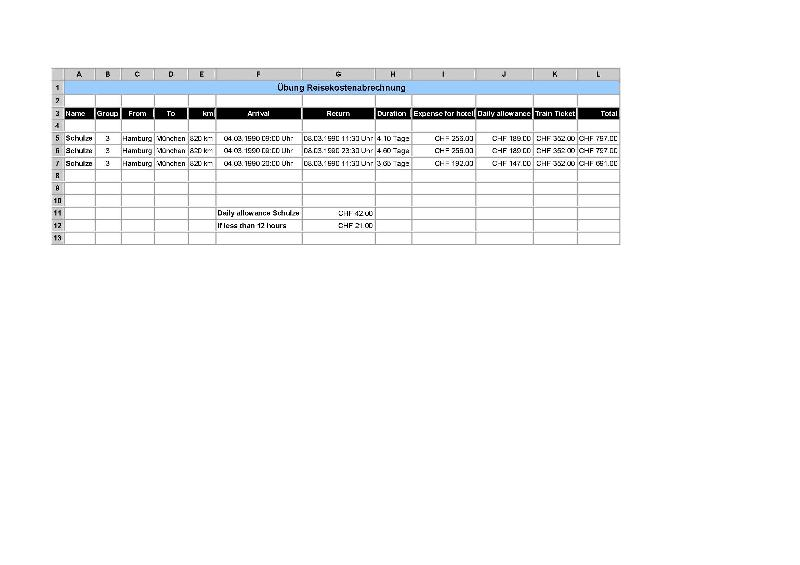# How to calculate daily allowance with Excel 2002Massimo Scola used Ask the Experts™
on
Hello everyone

I'm learning Excel and I find it very exciting because it can challenging. However, I sometimes do have difficulties like in the following exercise. I need to calculate the daily allowance of an employee. Please note that the names and titles are in German because I'm in Switzerland. I use Excel 2003.

Here is my question: If an employee stays for more than 12 hours, he will get the full daily allowance, otherwise he will only get half. The day starts at 0:00 (midnight). So if an employee arrives at 11:00, he will receive the full daily allowance - as he will stay there for 13 hours. If he arrives at 13:00, he will only get half because he stays for less than 12 hours on that day.

Please check with my Excel file, rows 5,6 and 7. I have used the same employee but the arrivals and departures are different. I have used the same employee because he gets the same daily allowance and it is easy to compare. The daily allowance will be in column J. I used the following method to calculate the allowance in column J, which proofed to be wrong:

H5 = Duration =  G5 - F5

J5 = Allowance = =TRUNC(H5)*42+(MOD(H5;1)>0)*21

I would appreciate it very much if someone could help me solve this problem, by finding the correct formula in J5.

Thanks a lot

MassimoQuestion-20.10.2010.xls
Comment
Watch Question

Do more withEXPERT OFFICE® is a registered trademark of EXPERTS EXCHANGE®
Expert of the Quarter 2010
Expert of the Year 2010

Commented:
Try this?

=(ROUND(DAUER*2,0))/2*
IF(Tarifgruppe=1,46,
IF(Tarifgruppe=2,44,
IF(Tarifgruppe=3,42,
IF(Tarifgruppe=4,40))))
J5 ...  =IF(H5>=12,H5*42,H5*21)

Hard to follow some of the names but I am guessing that Duration is the hours worked?  If so then just use the hours worked by 12 hours or more and multiply by 42 (Again guessing that is the allowance) and if it is less than 12 multiply the hours worked by 21.

Copy and paste that down the column of J5 ... J7 ... J99 whatever and it should work.
See attached file.
I included two columns to determine the number of full days and the number of half days. I assume that for both the arrival and departure days you would need to determine wether or not fullday allowance is required.
I adjusted the formula that calculates the allowance, replace the 4 nested if statements with the choose function. Just to show you an alternative.
Regards,
Egbert

Question-20.10.2010-1-.xls
Most Valuable Expert 2011
Awarded 2010
Commented:
Hello,

you can use the Ceiling() function

4.10 Tage       =CEILING(H5,0.5)      = 4.5
4.60 Tage       =CEILING(H6,0.5)      = 5
3.65 Tage       =CEILING(H7,0.5)      = 4

Then multiply that by the Tarifgruppe

=CEILING(H5,0.5)*IF(Tarifgruppe=1,46,IF(Tarifgruppe=2,44,IF(Tarifgruppe=3,42,IF(Tarifgruppe=4,40,"Fehler"))))

Instead of the nested IFs you can also use the Choose() function:

=CEILING(H5,0.5)*CHOOSE(Tarifgruppe,46,44,42,40)

cheers, teylyn

Most Valuable Expert 2011
Awarded 2010

Commented:
@JollyECS, good point about the half days for arrival and departure.
Most Valuable Expert 2011
Awarded 2010

Commented:
I think 33962216 Author:JollyECS nailed it.
Thanks teylyn.
I liked your 'CEILING'. That one was new to me, so thanks for increasing my knowledge!
Expert of the Quarter 2010
Expert of the Year 2010
Commented:
OP's formula using MOD

J5 = Allowance = =TRUNC(H5)*42+(MOD(H5;1)>0)*21

Achieves the same as teylyn's CEILING.
Therefore the answer must be along the lines of JollyECS's half day calculations.
But to be fair, Jolly's formula had an error - see row 7, it should be 4 days (3+.5+.5) not 3 (2+.5+.5)

Assuming that I am right in the analysis above, the formula for days should be
=INT(G5)-INT(F5)-1+CEILING(1-MOD(F5;1),0.5)+CEILING(MOD(G5;1),0.5)

and the allowance either (hardcoded, borrowing teylyn's formula)

=(INT(G5)-INT(F5)-1+CEILING(1-MOD(F5;1),0.5)+CEILING(MOD(G5;1),0.5))*CHOOSE(Tarifgruppe;46;44;42;40)

or (using the table in Mitarbeiter directly)

=(INT(G5)-INT(F5)-1+CEILING(1-MOD(F5;1),0.5)+CEILING(MOD(G5;1),0.5))*VLOOKUP(Tarifgruppe;Mitarbeiter!D1:F5;3;FALSE)

Not sure if this is relevant, but it appears the OP is using ; as the delimiter, which may be why none of the formulas worked (even if they did what the posters wanted).
VBA Developer

Commented:
This question has been classified as abandoned and is being closed as part of the Cleanup Program.  See my comment at the end of the question for more details.

Do more withSubmit tech questions to Ask the Experts™ at any time to receive solutions, advice, and new ideas from leading industry professionals.